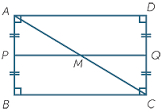Rectangle - area from WL

What is the area of a rectangle that is 1/2 of a meter long and 3/8 of meter wide?

A =  0.1875 m2

Step-by-step explanation:Did you find an error or inaccuracy? Feel free to write us. Thank you!Tips to related online calculators
Need help to calculate sum, simplify or multiply fractions? Try our fraction calculator.
Do you want to convert length units?

You need to know the following knowledge to solve this word math problem:

We encourage you to watch this tutorial video on this math problem: